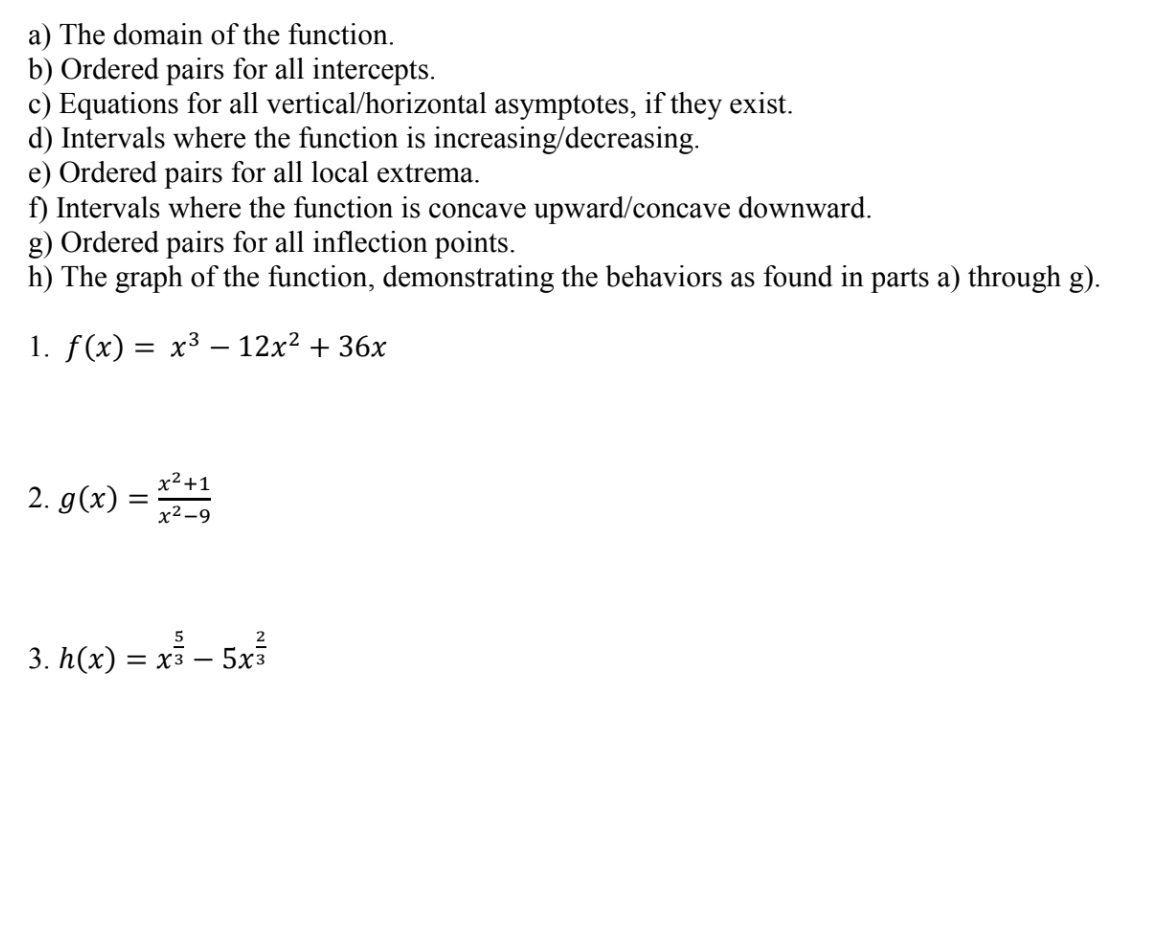# a) The domain of the function.b) Ordered pairs for all intercepts.c) Equations for all vertical/horizontal asymptotes, if they existd) Intervals where the function is increasing/decreasinge) Ordered pairs for all local extremaft) Intervals where the function is concave upward/concave downwardg) Ordered pairs for all inflection points.h) The graph of the function, demonstrating the behaviors as found in parts a) through g)I. f(x)-x3-12x2 + 36X2x2-9523. h(x) -xs - 5x3

Question
390 views

Hi, I keep submitting the same question regarding Q#3 f to H and landed all 3 different answers on Bartleby. Some experts said the inflection point is (-1,-6) while others got (-1.5,-8.52). Also for the concavity as well. I got one answer that was concave up on (-1.5,0)U(0,inf) and function is concave down on (-inf, -1.5),  concave upward on  (-1, 0) and concave downward on (- ∞, -1)  and (0, ∞), and concave downwards in the interval (−∞, −1) and concave upwards in the interval (−1, ∞). I do not know which was is correct since they're all different. Please help me clarify this issue as it affects my graph. Thank youhelp_outlineImage Transcriptionclosea) The domain of the function. b) Ordered pairs for all intercepts. c) Equations for all vertical/horizontal asymptotes, if they exist d) Intervals where the function is increasing/decreasing e) Ordered pairs for all local extrema ft) Intervals where the function is concave upward/concave downward g) Ordered pairs for all inflection points. h) The graph of the function, demonstrating the behaviors as found in parts a) through g) I. f(x)-x3-12x2 + 3 6X 2 x2-9 5 2 3. h(x) -xs - 5x3 fullscreen
check_circle

star
star
star
star
star
1 Rating
Step 1

Since, you have requested for question 3 part f to h, we will solve these parts of the solution.

Step 2

First find the interval for the function h(x)=x^(5/3)-5x^(2/3) where the function is concave upward or concave downward.

Step 3

The above derivative of the function is undefined at x=0. The graph of the break at the point x=0 and x=-1 into three interval which is as (-inf, -1), (-1, 0) and (0, -inf). Now, check the value of second derivative for particular point in the int...

### Want to see the full answer?

See Solution

#### Want to see this answer and more?

Solutions are written by subject experts who are available 24/7. Questions are typically answered within 1 hour.*

See Solution
*Response times may vary by subject and question.
Tagged in

### Calculus